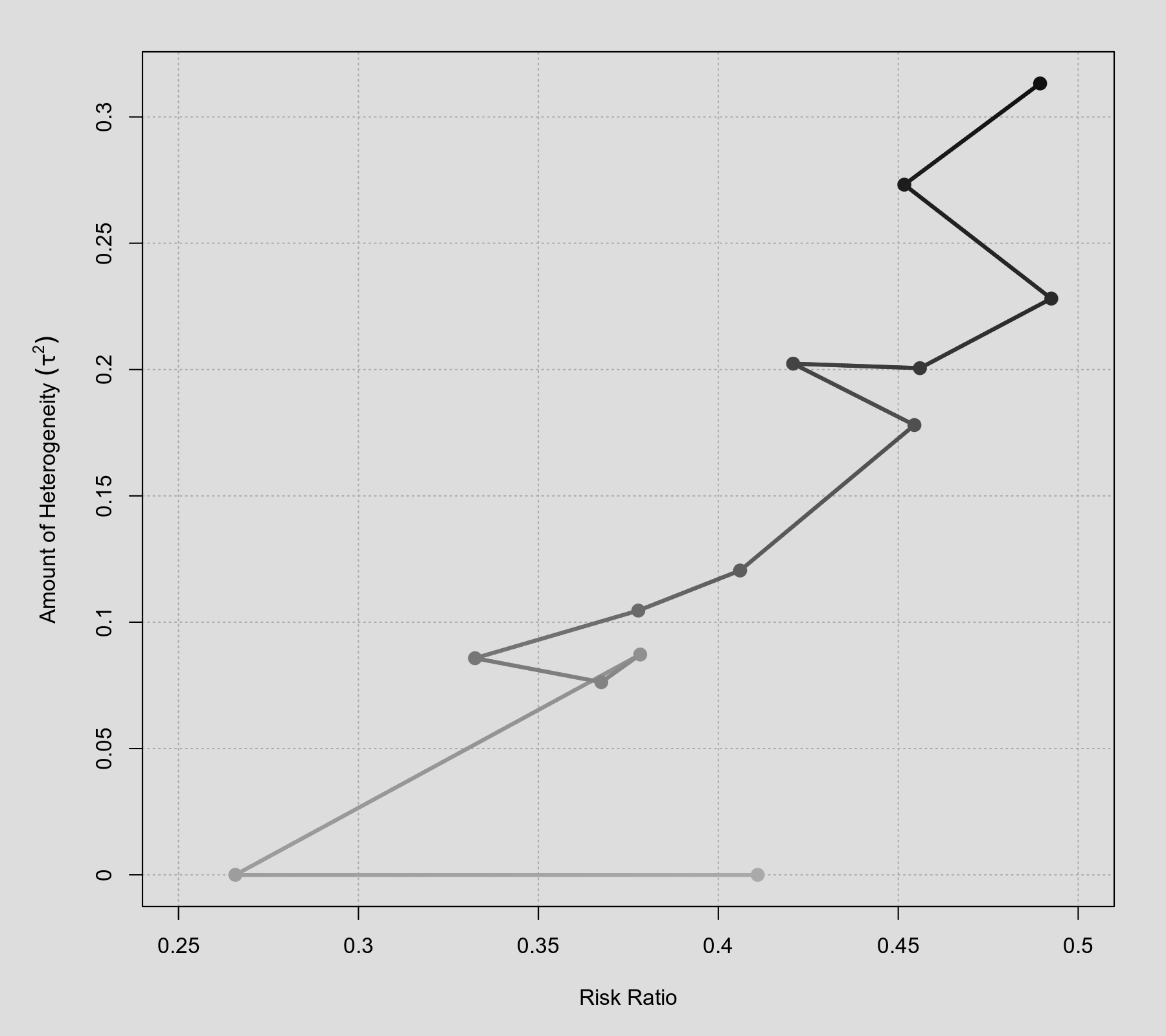#The metafor Package

A Meta-Analysis Package for R

### Sidebar

plots:plot_of_cumulative_results

## Plot of Cumulative Results

### Description

Instead of using a cumulative forest plot, another way to illustrate the results from a cumulative meta-analysis is to plot the estimate of the average effect against the estimated amount of heterogeneity as each study is added to the analysis in turn. An object returned by the cumul() function can be passed to the plot() function, which will then draw such a plot. The color gradient of the points/lines indicates the order of the cumulative results (by default, light gray at the beginning, dark gray at the end).

### Plot### Code

library(metafor)

### calculate (log) risk ratios and corresponding sampling variances
dat <- escalc(measure="RR", ai=tpos, bi=tneg, ci=cpos, di=cneg, data=dat.bcg)

### fit random-effects models
res <- rma(yi, vi, data=dat)

### cumulative meta-analysis (in the order of publication year)
tmp <- cumul(res, order=order(dat\$year))

### plot of cumulative results
plot(tmp, transf=exp, xlim=c(0.25,0.5), lwd=3, cex=1.3)